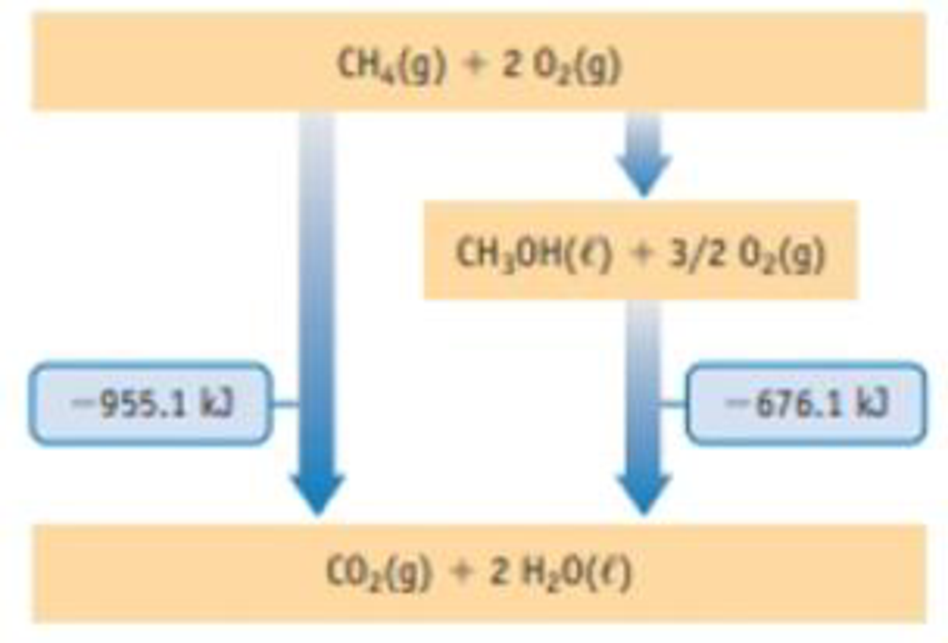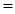# Methane, CH 4 , can be converted to methanol, which, like ethanol, can be used as a fuel. The energy level diagram shown here presents relationships between energies of the fuels and their oxidation products. Use the information in the diagram to answer the following questions. (The energy terms are per mol-rxn.) (a) Which fuel, methanol or methane, yields the most energy per mole when burned? (b) Which fuel yields the most energy per gram when burned? (c) What is the enthalpy change for the conversion of methane to methanol by reaction with O 2 (g)? (d) Each arrow on the diagram represents a chemical reaction. Write the equation for the reaction that converts methane to methanol.### Chemistry & Chemical Reactivity

9th Edition
John C. Kotz + 3 others
Publisher: Cengage Learning
ISBN: 9781133949640

#### Solutions

Chapter
Section### Chemistry & Chemical Reactivity

9th Edition
John C. Kotz + 3 others
Publisher: Cengage Learning
ISBN: 9781133949640
Chapter 5, Problem 115SCQ
Textbook Problem
1 views

## Methane, CH4, can be converted to methanol, which, like ethanol, can be used as a fuel. The energy level diagram shown here presents relationships between energies of the fuels and their oxidation products. Use the information in the diagram to answer the following questions. (The energy terms are per mol-rxn.)(a) Which fuel, methanol or methane, yields the most energy per mole when burned? (b) Which fuel yields the most energy per gram when burned? (c) What is the enthalpy change for the conversion of methane to methanol by reaction with O2(g)? (d) Each arrow on the diagram represents a chemical reaction. Write the equation for the reaction that converts methane to methanol.

(a)

Interpretation Introduction

Interpretation:

The fuel that yields the most energy per mole when burned has to be determined

Concept Introduction:

Heat energy required to raise the temperature of 1g of substance by 1K.Energy gained or lost can be calculated using the below equation.

q=C×m×ΔT

Where, q= energy gained or lost for a given mass of substance (m), C =specific heat capacity,ΔT= change in temperature.

The standard molar enthalpy of formation is the enthalpy change ΔfH0 is the enthalpy change for the formation of 1mol of a compound directly from its component elements in their standard states. And is given by

ΔrH0ΣnΔfH0(products)-ΣnΔfH0(reactants)

### Explanation of Solution

From the energy level diagram,

Methane liberates at 955.1kJ/mol

Methanol libe

(b)

Interpretation Introduction

Interpretation:

The fuel yields the most energy per gram when burned has to be calculated.

Concept Introduction:

Heat energy required to raise the temperature of 1g of substance by 1K.Energy gained or lost can be calculated using the below equation.

q=C×m×ΔT

Where, q= energy gained or lost for a given mass of substance (m), C =specific heat capacity,ΔT= change in temperature.

The standard molar enthalpy of formation is the enthalpy change ΔfH0 is the enthalpy change for the formation of 1mol of a compound directly from its component elements in their standard states. And is given by

ΔrH0ΣnΔfH0(products)-ΣnΔfH0(reactants)

(c)

Interpretation Introduction

Interpretation:

The enthalpy change for the conversion of methane by reaction with O2(g) has to be determined.

Concept Introduction:

Heat energy required to raise the temperature of 1g of substance by 1K. Energy gained or lost can be calculated using the below equation.

q=C×m×ΔT

Where, q= energy gained or lost for a given mass of substance (m), C =specific heat capacity, ΔT= change in temperature.

The standard enthalpy change of combustion of a compound is the enthalpy change which occurs when one gram of the compound is burned completely in oxygen under standard conditions, and with everything in its standard state.

ΔrH0=ΣnΔfH0(products)-ΣnΔfH0(reactants)

(d)

Interpretation Introduction

Interpretation:

The equation for the reaction that converts methane to methanol has to be identified.

Concept Introduction:

Heat energy required to raise the temperature of 1g of substance by 1K. Energy gained or lost can be calculated using the below equation.

q=C×m×ΔT

Where, q= energy gained or lost for a given mass of substance (m), C =specific heat capacity, ΔT= change in temperature.

The standard enthalpy change of combustion of a compound is the enthalpy change which occurs when one gram of the compound is burned completely in oxygen under standard conditions, and with everything in its standard state.

ΔrH0=ΣnΔfH0(products)-ΣnΔfH0(reactants)

### Still sussing out bartleby?

Check out a sample textbook solution.

See a sample solution

#### The Solution to Your Study Problems

Bartleby provides explanations to thousands of textbook problems written by our experts, many with advanced degrees!

Get Started

Find more solutions based on key concepts
How might nutritional genomics influence health care in the future?

Understanding Nutrition (MindTap Course List)

Cells a. are self-contained, living units. b. serve the bodys needs but have few needs of their own. c. remain ...

Nutrition: Concepts and Controversies - Standalone book (MindTap Course List)

In the following diagram, designate each daughter cell as diploid (2n) or haploid (n).

Human Heredity: Principles and Issues (MindTap Course List)

A student claims that the second of two lightbulbs in series is less bright than the first because the first li...

Physics for Scientists and Engineers, Technology Update (No access codes included)

Determine the number of seconds in 15 hours.

Introductory Chemistry: An Active Learning Approach

What feature is unique to cnidarians? Name some cnidarians.

Oceanography: An Invitation To Marine Science, Loose-leaf Versin